×#### Thank you for registering.

One of our academic counsellors will contact you within 1 working day.

Click to Chat

1800-1023-196

+91-120-4616500

CART 0

• 0

MY CART (5)

Use Coupon: CART20 and get 20% off on all online Study Material

ITEM
DETAILS
MRP
DISCOUNT
FINAL PRICE
Total Price: Rs.

There are no items in this cart.
Continue Shopping• Complete JEE Main/Advanced Course and Test Series
• OFFERED PRICE: Rs. 15,900
• View Details

```Chapter 27 Introduction to Graphs Exercise 27.2

Question: 1

The following table shows the number of patients discharged from a hospital with HIV diagnosis in different years:

Years:
2002
2003
2004
2005
2006

Number of Patients:
150
170
195
225
230

Represent this information by a graph.

Solution:

Here, year is an independent variable and the number of patients is a dependent variable. So, we take years on the x-axis and the number of patients on the y-axis.

Let us choose the following scale:

On x-axis: 2 cm = 1 year

On y-axis: 1 cm = 10 patients

Also, let us assume that on the x-axis, origin (O) represents 2001 and on the y-axis, origin (O) represents 120, i.e. O (2001, 120).

Now, let us plot (2002, 150), (2003, 170), (2004, 195), (2005, 225), (2006, 230). These points are joined to get the graph representing the given information as shown in the figure below.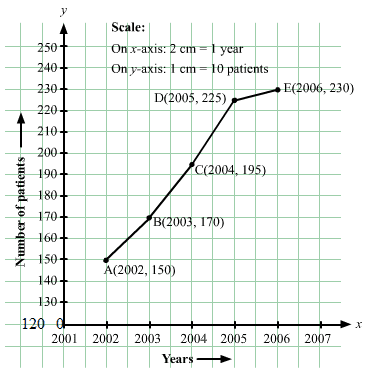Question: 2

The following table shows the amount of rice grown by a farmer in different years:

Years:
2000
2001
2002
2003
2004
2005
2006

Rice grown (in quintals):
200
180
240
260
250
200
270

Plot a graph to illustrate this information.

Solution:

Here, the year is an independent variable and quantity of rice grown is a dependent variable. So, we take years on the x-axis and quantity of rice grown on the y-axis.

Let us choose the following scale:

On x-axis: 2 cm = 1 year

On y-axis: 1 cm = 20 quintals

Let us assume that the origin O represents the coordinates (1999, 160).

Now, let us plot (2000, 200), (2001, 180), (2002, 240), (2003, 260), (2004, 250),(2005, 200),(2006, 270).

These points are joined to get the graph representing the given information as shown in the figure below.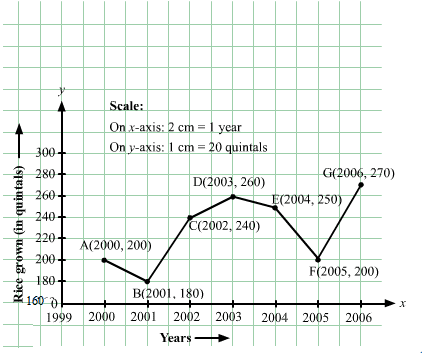Question: 3

The following table gives the information regarding the number of persons employed to a piece of work and time taken to complete the work:

Number of persons:
2
4
6
8

Time taken (in days):
12
6
4
3

Plot a graph of this information.

Solution:

Here, the number of persons is an independent variable and time taken is a dependent variable. So, we take the number of persons on the x-axis and time taken on the y-axis.

Let us choose the following scale:

On x-axis: 2 cm = 2 persons

On y-axis: 2 cm = 2 days

Now, let us plot (2, 12), (4, 6), (6, 4), (8, 3). These points are joined to get the graph representing the given information as shown in the figure below.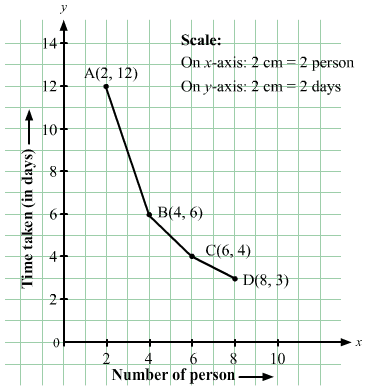Question: 4

The following table gives the information regarding the length of a side of a square and its area:

Length of a side (in cm):
1
2
3
4
5

Area of square (in cm2)
1
4
9
16
25

Draw a graph to illustrate this information.

Solution:

Here, length of a side is an independent variable and area of the square is a dependent variable. So, we take the length of a side on the x-axis and area of the square on the y-axis.

Let us choose the following scale:

On x-axis: 2 cm = 1 cm

On y-axis: 1 cm = 2 cm2

Now we plot (1,1), (2,4), (3,9), (4,16), (5,25). These points are joined to get the graph representing the given information as shown in the figure below.Question: 5

The following table shows the sales of a commodity during its years 2000 to 2006.

Years:
2000
2001
2002
2003
2004
2005
2006

Sales (in lakhs of Rs):
1.5
1.8
2.4
3.2
5.4
7.8
8.6

Draw a graph of this information.

Solution:

Here, year is an independent variable and sales is a dependent variable. So, we take year on the x-axis and sales on the y-axis.

Let us choose the following scale:

On x-axis: 2 cm = 1 year

On y-axis: 2 cm = 1 lakh rupees

Assume that on x-axis, origin (O) represents 1991.

So, the coordinates of O are (1991,0).

Now, let us plot (2000, 1.5), (2001, 1.8), (2002, 2.4), (2003, 3.2), (2004, 5.4), (2005, 7.8) and (2006, 8.6). These points are joined to get the graph representing the given information as shown in the figure below.Question: 6

Draw the temperature-time graph in each of the following cases:

(i)

Time (in hours):
7:00
9:00
11:00
13:00
15:00
17:00
19:00
21:00

Temperature (°F) in:
100
101
104
102
100
99
100
98

(ii)

Time (in hours):
8:00
10:00
12:00
14:00
16:00
18:00
20:00

Temperature (°F) in:
100
101
104
103
99
98
100

Solution:

Here, time is an independent variable and temperature is a dependent variable. So, we take time on the x-axis and temperature on the y-axis.

Let us choose the following scale:

For point (i):

On x-axis: 1 cm = 1 hours

On y-axis: 1 cm = 2°F

For point (ii):

On x-axis: 2 cm = 2 hours

On y-axis: 1 cm = 1°F

Let us assume that on the x-axis, the coordinate of origin is 6:00.

On y-axis, the coordinate of origin is 94°F .

So, the coordinates of 0 are (6:00, 94).

Now, let us plot (7:00, 100), (9:00, 101), (11:00, 104),..(21:00, 98) for point (i) and (8:00, 100), (10:00, 101), (12:00, 104) ….. (20:00, 100) for point (ii). These points are joined to get the graphs representing the given information as shown in the figures below.

(i)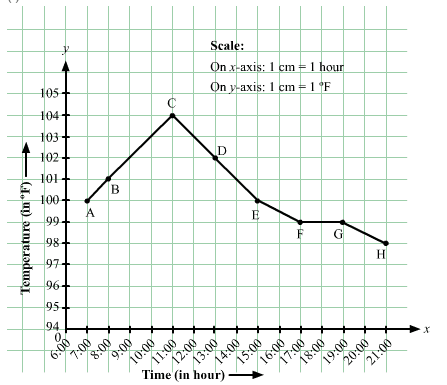(ii)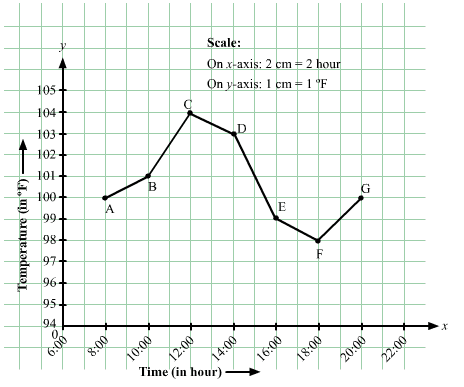Question: 7

Draw the velocity-time graph from the following data:

Time (in hours):
7:00
8:00
9:00
10:00
11:00
12:00
13:00
14:00

Speed (in km/hr):
30
45
60
50
70
50
40
45

Solution:

Here, time is an independent variable and speed is a dependent variable. So, we take time on the x-axis and speed on the y-axis.

Let us choose the following scale:

On x-axis: 2 big division = 1 hour

On y-axis: 1 big division = 10 km/hr

Let us assume that on the x-axis, the coordinate of origin (O) is 7:00.

So, the coordinates of O are (7:00,0).

Now, let us plot (7:00,30), (8:00,45), (9:00,60), (10:00,50), (11:00,70), (12:00,50), (13:00,40), (14:00,45). These points are joined to get the graph representing the given information as shown in the figure below.Question: 8

The runs scored by a cricket team in first 15 overs are given below:

Overs:
I
II
III
IV
V
VI
VII
VIII
IX
X
XI
XII
XIII
XIV
XV

Runs:
2
1
4
2
6
8
10
21
5
8
3
2
6
8
12

Draw the graph representing the above data in two different ways as a graph and a bar chart.

Solution:

Here, over is an independent variable and run is a dependent variable. So, we takeovers on the x-axis and runs the on y-axis.

Let us choose the following scale:

On x-axis: 1 cm = 1 over

On y-axis: 1 cm = 2 runs

Now, let us plot (I,2), (II,1), (III,4) (XV,12). These points are joined to get the graph representing the given information as shown in the figure below.Question: 9

The runs scored by two teams A and B in first 10 overs are given below:

Overs:
I
II
III
IV
V
VI
VII
VIII
IX
X

Team A:
2
1
8
9
4
5
6
10
6
2

Team B:
5
6
2
10
5
6
3
4
8
10

Draw a graph depicting the data, making the graphs on the same axes in each case in two different ways as a graph and as a bar chart.

Solution:

Here, over is an independent variable and run is a dependent variable. So, we takeovers on x-axis and runs on y-axis.

Let us choose the following scale:

On x-axis: 1 cm = 1 over

On y-axis: 1 cm = 1 run

Now, let us plot (I,2), (II,1), (III,8) (X,2) for team A and (I,5), (II,6), (III,8) ….. (X,10) for team B. These points are joined to get the graph representing the given information as shown in the figure below.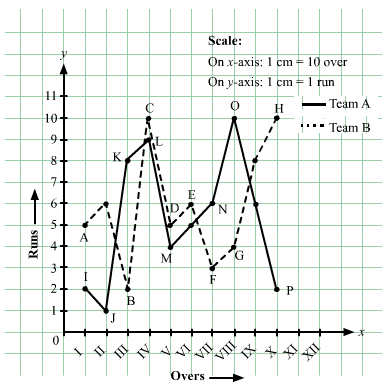```### Course Features

• 728 Video Lectures
• Revision Notes
• Previous Year Papers
• Mind Map
• Study Planner
• NCERT Solutions
• Discussion Forum
• Test paper with Video Solution